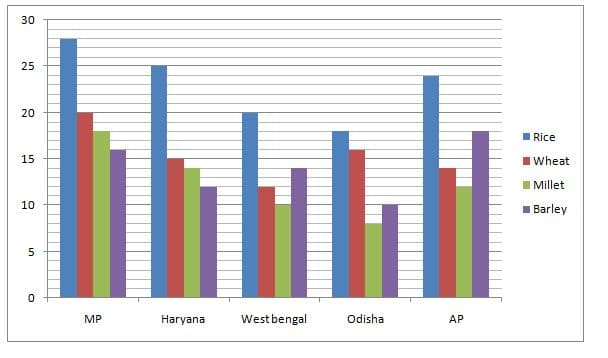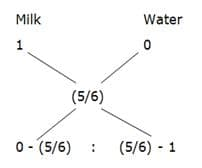# LIC Assistant Mains Quantitative Aptitude Questions 2019 – (Day-4)

Dear Aspirants, Our IBPS Guide team is providing new pattern Quantitative Aptitude Questions for LIC Assistant Mains 2019 so the aspirants can practice it on a daily basis. These questions are framed by our skilled experts after understanding your needs thoroughly. Aspirants can practice these new series questions daily to familiarize with the exact exam pattern and make your preparation effective.

Check here for LIC Assistant Mains Mock Test 2019

[WpProQuiz 7523]

Application Sums

1) In what ratio water be mixed with milk to earn a profit of 20 % by selling the mixture at cost price?

a) 8: 5

b) 11: 9

c) 9: 5

d) 7: 4

e) 5: 1

2) A box contains x red balls and 5 pink balls. If the probability of taking 2 pink balls is 5/33, then find the number of red balls?

a) 4

b) 8

c) 7

d) 6

e) 5

3) Incomes of Rahul and Kathir are in the ratio 4 : 5 and their expenditures are in the ratio 6 : 7. If Rahul and Kathir saves Rs. 4000 and Rs. 8000 respectively, then find the income of Rahul?

a) Rs. 44000

b) Rs. 36000

c) Rs. 40000

d) Rs. 32000

e) None of these

4) A Boat takes 2 hours less to travel to 80 Km downstream than to travel the same distance upstream. If the speed of the stream is 10 Km/hr, then find the speed of boat in still water?

a) 32 km/hr

b) 24 km/hr

c) 36 km/hr

d) 30 km/hr

e) None of these

5) How many 4 letter words with or without meaning can be formed out of the letters of the word, “ENORMITY”, if repetition of letters is not allowed?

a) 380

b) 2840

c) 1680

d) 5660

e) None of these

Data Interpretation

Directions (Q. 6 – 10): Study the following information carefully and answer the given questions?

The following bar graph shows the total production (In thousand tonnes) of Rice, Wheat, Millet and Barley in different states.6) Find the difference between the average productions of Rice to that of Barley in all the given states together?

a) 9 thousand tonnes

b) 11 thousand tonnes

c) 6 thousand tonnes

d) 15 thousand tonnes

e) None of these

7) Find the ratio between the total production of all the given food grains in MP to that of West Bengal?

a) 35 : 19

b) 41 : 28

c) 55 : 27

d) 49 : 53

e) None of these

8) Total production of Millet in MP, Haryana and West Bengal together is approximately what percentage of total production of Wheat in Haryana, Odisha and AP together?

a) 65 %

b) 80 %

c) 95 %

d) 110 %

e) 125 %

9) The total production of all the given food grains in Odisha is approximately what percentage more/less than the total production of all the given food grains in AP?

a) 25 % less

b) 40 % more

c) 25 % more

d) 40 % less

e) 55 % more

10) Find the difference between the total production of all the given food grains in Haryana to that of total production of Wheat in all the given states together?

a) 6 thousand tonnes

b) 14 thousand tonnes

c) 9 thousand tonnes

d) 11 thousand tonnes

e) None of these

Directions (1-5):

Let the cost price of milk be Rs. 1

Selling price of mixture = Rs. 1

Cost price of mixture = 1 * 100/120 = 5/6= > – (5/6) : – (1/6)

= > 5 : 1

Let the number of red balls be x,

Probability = 5C2/(x+5)C2 =  5/33

(5 * 4) /[(x + 5)(x + 4)]= 5/33

132 = (x + 5) (x + 4)

x2 + 9x + 20 = 132

x2 + 9x – 112 = 0

(x + 16) (x – 7) = 0

x = -16, 7 (Negative value will be eliminated)

So, x = 7

The number of red balls = 7 balls

Incomes of Rahul and Kathir are in the ratio = 4 : 5 (4x, 5x)

According to the question,

= > (4x – 4000)/(5x – 8000) = 6/7

= > 28x – 28000 = 30x – 48000

= > 2x = 20000

= > x = 10000

Income of Rahul = 4x = Rs. 40000

Let the speed of boat in still water be x,

According to the question,

2 = 80/(x – 10) – 80/(x + 10)

2 = 80 * [1/(x – 10) – 1/(x + 10)]

1 = 40 * [(x + 10 – x + 10) / (x2 – 100)]

x2 – 100 = 40 * 20

x2 = 800 + 100 = 900

x = 30

The speed of boat in still water = 30 km/hr

“ENORMITY”, contains 8 different letters.

Required number of words = 8p4

= > (8 * 7 * 6 * 5) = 1680

Directions (6-10):

The average production of Rice in all the given states together

= > (28 + 25 + 20 + 18 + 24) / 5

= > 115 / 5 = 23 thousand tonnes

The average production of Barley in all the given states together

= > (16 + 12 + 14 + 10 + 18) / 5

= > 70 / 5 = 14 thousand tonnes

Required difference = 23 – 14 = 9 thousand tonnes

The total production of all the given food grains in MP

= > 28 + 20 + 18 + 16 = 82 thousand tonnes

The total production of all the given food grains in West Bengal

= > 20 + 12 + 10 + 14 = 56 thousand tonnes

Required ratio = 82 : 56 = 41 : 28

Total production of Millet in MP, Haryana and West Bengal together

= > 18 + 14 + 10 = 42 thousand tonnes

Total production of Wheat in Haryana, Odisha and AP together

= > 15 + 16 + 14 = 45 thousand tonnes

Required % = (42 / 45) * 100 = 93.33 % = 95 %

The total production of all the given food grains in Odisha

= > 18 + 16 + 8 + 10 = 52 thousand tonnes

The total production of all the given food grains in AP

= > 24 + 14 + 12 + 18 = 68 thousand tonnes

Required % = [(68 – 52) / 68] * 100 = 23.52 % = 25 % less

The total production of all the given food grains in Haryana

= > 25 + 15 + 14 + 12 = 66 thousand tonnes

The total production of Wheat in all the given states together

= > 20 + 15 + 12 + 16 + 14 = 77 thousand tonnes

Required difference = 77 – 66 = 11 thousand tonnes